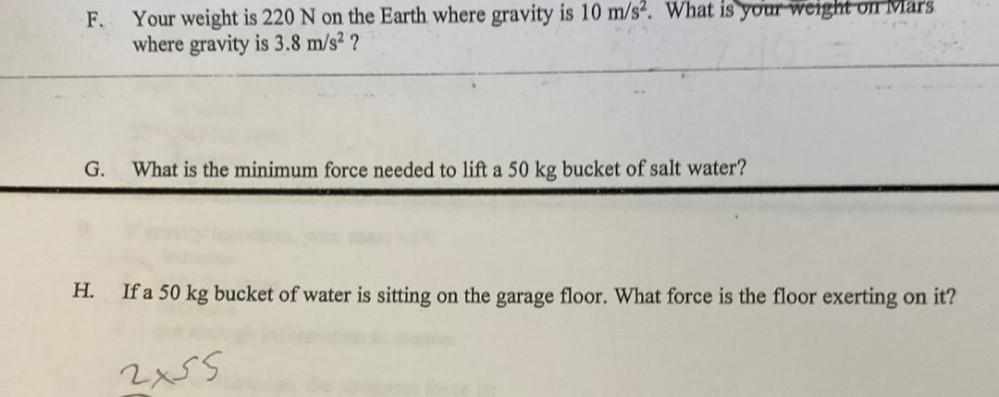Question:

# Your weight is 220 N on the Earth where gravity is 10 m/s². What is your weight on Mars where gravity is 3.8 m/s²?Your weight is 220 N on the Earth where gravity is 10 m/s². What is your weight on Mars where gravity is 3.8 m/s²?